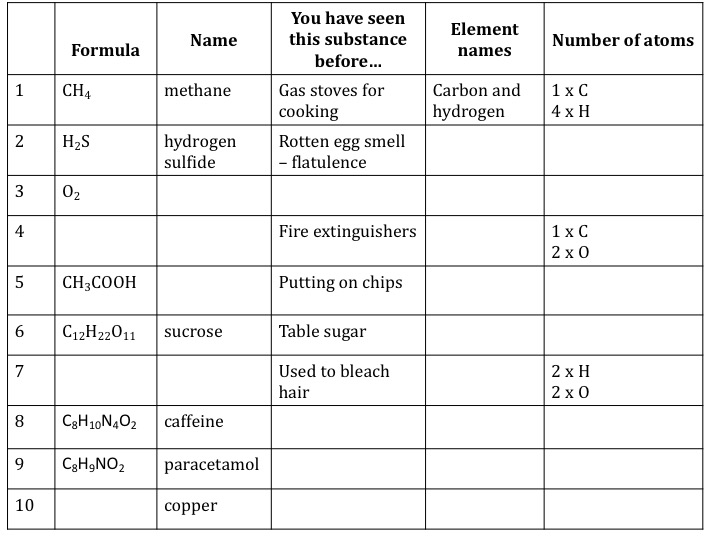# Writing chemical formulae teaching resources

###### Worksheets and lesson ideas to challenge students aged 11 to 16 to think hard about chemical formulae. (GCSE and Key Stage 3)
Writing chemical formula teacher brief

Overview: all matter is made from particles. We use the word particle and not atom because sometimes atoms lose or gain electrons to form ions. Each substance has a specific ratio of atoms (or ions) that can be represented by a chemical formula. From looking at the formula you can often work out the type of bonding within the substance. Ionic substances are made from metals and non-metals whereas covalent substances are made from non-metals. The molecular formula is the actual number of atoms in a molecule. The empirical formula of a compound is the simplest whole number ratio of atoms of each element present in a compound. For example the molecular formula of glucose is C6H12O6 whereas the empirical formula is CH2O. For ionic compounds we use empirical formulae.

Key concept: every chemical substance has a fixed proportion of atoms or ions that can be represented by a chemical formula.

Linked knowledge: states of matter; bonding; chemical changes; atomic structure

Misconception/error [scientific idea]:

## Teaching resources

#### Where to start?

Brainstorm all the chemical formulae that students already know. Students can work in groups to do this in 1 minute, writing down the formula and the name. You can then ask students to compare and contrast the formulae for methane and ethane. How are they similar and how are they different? A Venn diagram would work well here.

####Molecular formulae (basic)

Key Stage 3 worksheet on molecular formulae. This resource asks students to think about what a molecular formula tells us about a chemical substance. You will need to adapt this activity if you haven’t covered covalent bonding yet (slide 3 and 4). It is helpful to have some general discussion around methane before moving into the abstract world of representations. You may also want to do methane bubbles in this lesson but make sure you risk assess this carefully (PDF)

#### Chemical formulae (advanced) – what does it mean?

Key Stage 3 worksheet on chemical formulae. This activity is to check that students understand what a chemical formula means. Do they know the difference between Cl, 2Cl and Cl2? So often students get to age 16 and don’t understand the language of chemistry, which makes learning more rewarding concepts much harder. (PDF)

#### Empirical formula

I always used to struggle to think of a good way to introduce empirical formula to students – why do we care?! I then saw a lesson where students were asked to count sodium and chloride ions in a picture of a giant lattice structure. They wrote crazy formulas such as Na20Cl20. They were then shown another picture of a different lattice of sodium chloride, and asked to count the number of ions there – students could see that although the numbers of ions changed, the simplest ratio of chloride to sodium ions remained the same. Now you can introduce the idea of empirical formula – a formula determined by experimentation, hence the name. This serves as a simple but powerful starting point that provides a rationale for why we care about empirical formulas.